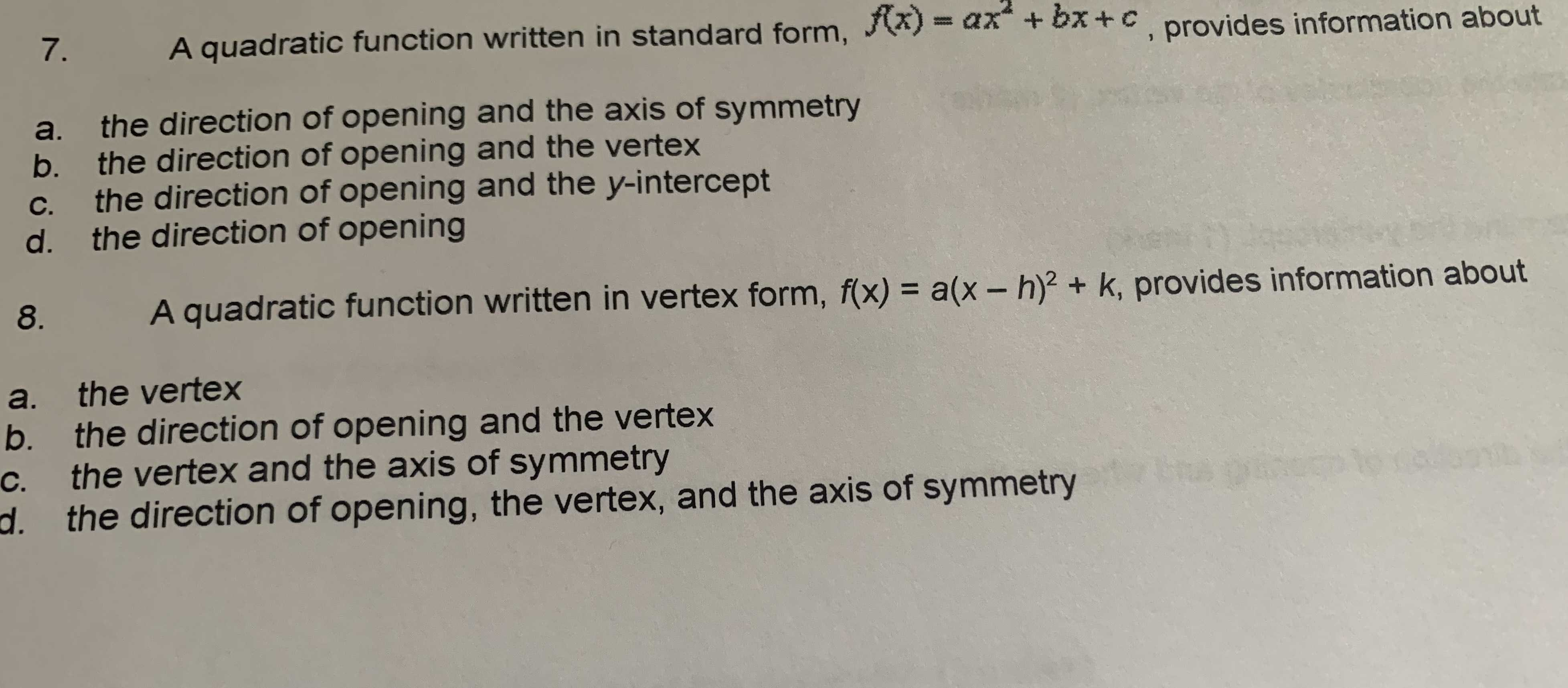### ¿Todavía tienes preguntas de matemáticas?

Pregunte a nuestros tutores expertos
Algebra
Pregunta7. A quadratic function written in standard form, $$f ( x ) = a x ^ { 2 } + b x + c$$ , provides information about

a. the direction of opening and the axis of symmetry

b. the direction of opening and the vertex

c. the direction of opening and the $$y$$ -intercept

d. the direction of opening

8. A quadratic function written in vertex form, $$f ( x ) = a ( x - h ) ^ { 2 } + k$$ , provides information about

a. the vertex

b. the direction of opening and the vertex

c. the vertex and the axis of symmetry

d. the direction of opening, the vertex, and the axis of symmetry